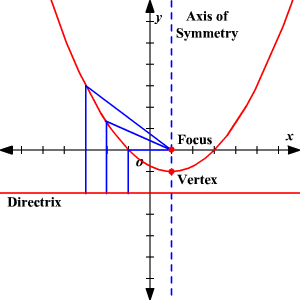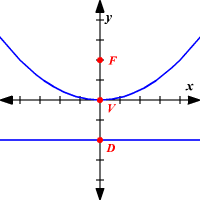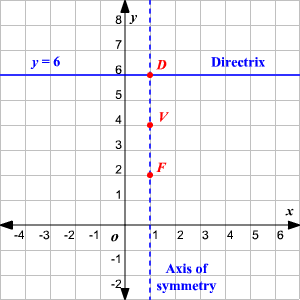# Directrix

A parabola is set of all points in a plane which are an equal distance away from a given point and given line. The point is called the focus of the parabola, and the line is called the directrix .

The directrix is perpendicular to the axis of symmetry of a parabola and does not touch the parabola. If the axis of symmetry of a parabola is vertical, the directrix is a horizontal line .If we consider only parabolas that open upwards or downwards, then the directrix is a horizontal line of the form $y=c$ .

### Relation between focus, vertex and directrix:

The vertex of the parabola is at equal distance between focus and the directrix.

If $F$ is the focus of the parabola, $V$ is the vertex and $D$ is the intersection point of the directrix and the axis of symmetry, then $V$ is the midpoint of the line segment $\stackrel{¯}{FD}$ .Example:

If a parabola has a vertical axis of symmetry with vertex at $\left(1,4\right)$ and focus at $\left(1,2\right)$ , find the equation of the directrix.

If $F$ is the focus of the parabola, $V$ is the vertex and $D$ is the intersection point of the directrix and the axis of symmetry, then $V$ is the midpoint of the line segment $\stackrel{¯}{FD}$ .

Equate the $x$ -coordinates and solve for $p$ .

$\begin{array}{l}1=\frac{1+p}{2}\\ 2=1+p\\ p=1\end{array}$

Equate the $y$ -coordinates and solve for $q$ .

$\begin{array}{l}4=\frac{2+q}{2}\\ 8=2+q\\ q=6\end{array}$

The equation of the directrix is of the form $y=c$ and it passes through the point $\left(1,6\right)$ . Here, $c=6$ .

So, the equation of the directrix is $y=6$ .

The graph is as shown.